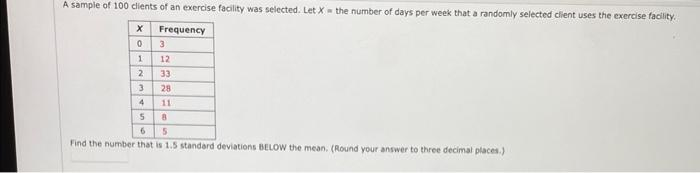Home / Expert Answers / Statistics and Probability / a-sample-of-100-clients-of-an-exercise-facility-was-selected-let-x-the-number-of-days-per-w-pa387

# (Solved): A sample of 100 clients of an exercise facility was selected. Let \( X= \) the number of days per w ...A sample of 100 clients of an exercise facility was selected. Let \( X= \) the number of days per week that a randomly selected client uses the cxercise facility. Find the number that is \( 1.5 \) standard deviations BeLow the mean. (Round your answer to three decimal places.)

We have an Answer from Expert